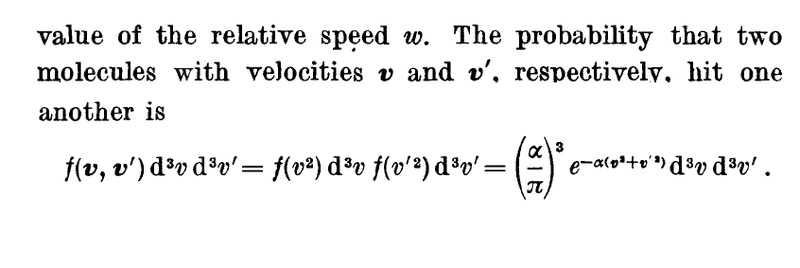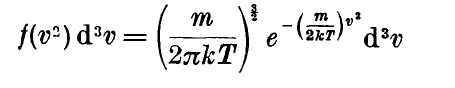Kinetic Theory of gases: pdf

Hi guys, I'm studying the Kinetic Theory of gases from Pauli's book Vol. 3. Here he describes a section on the Mean free path, where the probability of two particles with speed v and v' colliding is described as:What is f(v,v') here? Is it the velocity distribution function? If so, isn't it simply the fraction of molecules with velocities v and v' ?

Simon Bridge
Homework Helper
##f(\vec v, \vec v')## appears to be the probability that two molecules with velocities ##\vec v## and ##\vec v'## hit one another - as stated in the accompanying text.

It may be a bit confusing because it is expressed as a small range of velocities.

##f(\vec v, \vec v')## appears to be the probability that two molecules with velocities ##\vec v## and ##\vec v'## hit one another - as stated in the accompanying text.

It may be a bit confusing because it is expressed as a small range of velocities.

How did they get the exponential expression for f(v2) and f(v'2)?

And it is implied that the probability of both of them colliding is the product: f(v2) f(v'2) dv dv'

Then on its own, what does f(v2)dv and f(v'2)dv' mean?

Simon Bridge
Homework Helper
replace the "f"'s with "p"'s ... you may be able to read it better.

$$p(\vec v^2) = \sqrt{\frac{\alpha^3}{\pi}}e^{-\alpha \vec v^2}$$ ... comes from the distribution of kinetic energies perhaps (as ##K\propto v^2##) ...

You may find the following approach easier:
http://physics.bu.edu/~redner/542/refs/reif-chap12.pdf

replace the "f"'s with "p"'s ... you may be able to read it better.

$$p(\vec v^2) = \sqrt{\frac{\alpha^3}{\pi}}e^{-\alpha \vec v^2}$$ ... comes from the distribution of kinetic energies perhaps (as ##K\propto v^2##) ...

You may find the following approach easier:
http://physics.bu.edu/~redner/542/refs/reif-chap12.pdf

I have verified that the book meant f(v2) represents the fraction of molecules traveling with velocity v:Why is the product of f(v) and f(v') the probability that both particles with velocities v and v' collide? Technically the product means fraction of molecules with velocities v and v', implying a molecule having two velocities at the same time --- which doesn't make sense?

Simon Bridge
Homework Helper